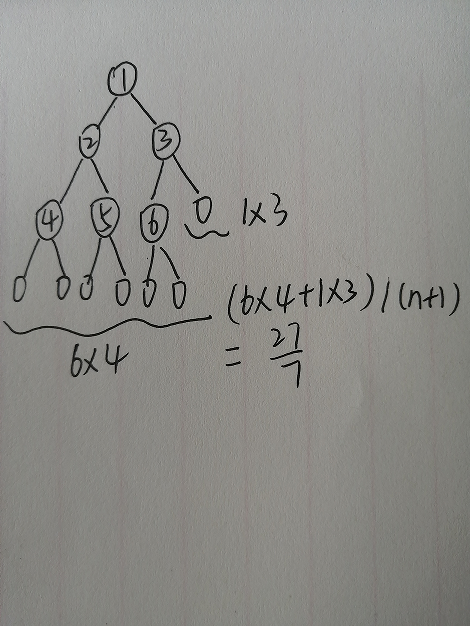• 2020-04-29 09:42:05

# 问题描述

对于长度为 9 9 的顺序存储的有序表 1 , 2 , 3 , 4 , 5 , 6 , 7 , 8 , 9 {1, 2, 3, 4, 5, 6, 7, 8, 9}算法 algorithm 数据结构
更多相关内容
• 二分查找平均查找长度二分查找平均查找长度进行简单分析。 向作出假设：要查找的元素在数组内，数组长度为 nnn. 约定对长度为 nnn 的数组，平均查找长度为随机变量 CnC_nCn​，随机变量 InI_nIn​ 定义如下 ...

# 二分查找的平均查找长度

对二分查找的平均查找长度进行简单分析。

向作出假设：要查找的元素在数组内，数组长度为 n n . 约定对长度为 n n 的数组，平均查找长度为随机变量 C n C_n ，随机变量 I n I_n 定义如下

I n = { 1 要 查 找 的 关 键 字 是 这 个 长 度 为 n 的 数 组 的 中 间 的 关 键 字 0 o t h e r w i s e , I_n=\left\{ \begin{matrix} 1 & 要查找的关键字是这个长度为 n 的数组的中间的关键字\\ 0 & \mathrm{otherwise} \end{matrix} \right.,

那么

C n = I n + ( 1 − I n ) ( C n / 2 + 1 ) , C_n=I_n+(1-I_n)(C_{n/2}+1),

取其期望值

E ( C n ) = 1 n + ( 1 − 1 n ) E ( C n / 2 + 1 ) < 1 n + 1 + E ( C n / 2 ) , E(C_n)=\frac1n+\left(1-\frac1n\right)E(C_{n/2}+1)<\frac1n+1+E(C_{n/2}),

展开

E ( C n ) = 1 + ⋯ + 2 ⌊ log ⁡ n ⌋ n + ⌊ log ⁡ n ⌋ + c = Θ ( log ⁡ n ) . E(C_n)=\frac{1+\cdots+2^{\lfloor\log{n}\rfloor}}n+\lfloor\log{n}\rfloor+c=\Theta(\log {n}).

简单分析，还有很多不完备的地方。

展开全文算法
• 编程统计，长度为N的有序数组进行二分查找时，查找成功与失败的平均查找长度。注意，表达成总查找长度除以总查找个数的形式。 输入样例: 输入数组的元素个数：一个正整数： 6 输出样例: 在第一行输出查找成功的平均...

#### 题意：

编程统计，长度为N的有序数组进行二分查找时，查找成功与失败的平均查找长度。注意，表达成总查找长度除以总查找个数的形式。

#### 输入样例:

输入数组的元素个数：一个正整数：

6

#### 输出样例:

在第一行输出查找成功的平均查找长度，在第二行输出查找失败的平均查找长度： （不要求计算，也不进行约分，仅输出成分数形式）

14/6
27/7

#### 例如：#### 代码：

#include<stdio.h>
#include<string.h>
#include<math.h>
#include<stdlib.h>
#include<queue>
#include<algorithm>
using namespace std;
int high(int n)
{
int s=0;
while(n)
{
n=n/2;
s++;
}
return s;
}
int main()
{
int n;
scanf("%d",&n);
int h=high(n);
int i,j,k;
int cg=0;
for(i=1; i<h; i++)
cg+=(i*pow(2,i-1));
int ss=0;
for(i=pow(2,h-1); i<=n; i++)
ss++;
cg+=(ss*h);
printf("%d/%d\n",cg,n);
int kk=ss*2*(h+1);
int mm=pow(2,h-1)-ss;
kk+=(mm*h);
printf("%d/%d\n",kk,n+1);
return 0;
}

展开全文• 设计一个程序，建立由有序序列R[0..n-1]进行二分查找产生的判定树，在此基础上完成如下功能： （1） 输出n=11时的判定树并求成功情况下的平均查找长度ASl （2） 通过构造判定树可以求得的成功情况下的平均查找长度...c++ 算法图片 算法
• 看数据结构书的时候碰上的内容，我自己将它化成关于级数的题，然后自己算的...满二叉树来分析折半查找平均长度   h=层高 n=节点数 []为计算过程的式   先算总查找次数 1*1+2*2+3*4+4*8...(h-1)*2^(h-2)+h*2...

来源：http://blog.csdn.net/turne/article/details/50488378

看数据结构书的时候碰上的内容，我自己将它化成关于级数的题，然后自己算的过程，基本就是等比级数和等差级数的混合内容。
满二叉树来分析折半查找的平均长度

h=层高 n=节点数
[]为计算过程的式

先算总查找次数
1*1+2*2+3*4+4*8...(h-1)*2^(h-2)+h*2^(h-1)  ………………

*2:

1*2+2*4+3*8+4*16...(h-1)*2^(h-1)+h*2^h  ……………………

-:
*2-=:
=:

-1*1-1*2-1*4-1*8-1*16...-2^(h-1)+h*2^h  ……………………… 

+h*2^h=…………………………………………………………………………[3.1]

-1*1-1*2-1*4-1*8-1*16...-2^(h-1) ……………………………………… 

*2-==

-2^h+1  …………………………………………………………………………………… 

因为=，所以把代入[3.1]可以得到下面的结果
=+h*2^h  =  -2^h+1+h*2^h=(h-1)*2^h+1

由于 (n+1=2^h)，所以有

=(n+1)log(n+1)-(n+1)+1
=(n+1)log(n+1)-n

最后，来求查找次数平均数
 /n = ((n+1)log(n+1)-n)/n

最终，平均查找长度约等于log(n+1)-1

上面的所有对数log的底数皆为2.
展开全文• 首先要明白，二分查找是建立在有序数组的基础上的。 二分查找主要有递归和非递归两种算法实现 /** * 二分查找的递归和非递归实现 * * @author ht * */ public class BinarySearch { public static void ...java实现
• 看数据结构书的时候碰上的内容，我...满二叉树来分析折半查找的平均长度二分查找二叉判定树 h=层高 n=节点数 []为计算过程的式 先算总查找次数 1*1+2*2+3*4+4*8...(h-1)*2^(h-2)+h*2^(h-1)  *2:图片 算法
• 查找： 给定一个值k，在含有n个元素的表中找出...平均查找长度（ASL）：平均需要比较的次数 ASL分为查找成功和查找失败两种情况 ASL越小，其时间性能越好 线性表的查找是一种最简单的查找表，分为顺序查..数据结构 算法 散列表
• 进行二分查找,等概率的情况下，查找成功的**平均查找长度平均比较次数）**是？？？ 可以建一颗二叉树 （本垃圾手绘图片） 鸭鸭鸭 观察一下，每次进行二分建树 （这很好理解对吧 ） 然后，重点来了 公式拿来，这个...c++
• 实验目的:* 深入掌握折半查找过程和折半查找算法分析* 实验内容:* 设计程序，建立有序序列R[0...n-1]进行二分查找产生的判断树，* 在此基础上完成如下功能:* 1、输出n=11时的判定树并求成功情况下的平均查找长度ASL...
• ASL(Average Search Length)，即平均查找长度，在查找运算中，由于所费时间在关键字的比较上，所以把平均需要和待查找值比较的关键字次数成为平均查找长度。它的定义是这样的：其中n为查找表中元素个数，Pi为查找第i...
• 在无序的数组中查找一指定值，必须遍历整个数组，直至查找成功，计算其平均查找次数也相当简单： avg线性 = (1 + n) / 2 因在数组中，各个元素被查找的概率相等，最好的情况是第1个就是目标元素；最坏的情况是...
• ​ 给定一个规模为n的按照数字从小到大排序的...1、计算数据规模为n二分查找的时间复杂度 循环次数 剩下的数据规模 第一次查找： T(1) = n/2 第二次查找： T(2) = n/2^2 第三次查找： T(3) = n/2^3 … 第M次查找： T(M)算法
• 建完全二叉树 例如长度为12的有序线性表，构建完全二叉树，如下图： 平均查找长度为：3.1
• Golang语言实现 实现二分查找，二分左侧查找，二分右侧查找，直接贴代码，其中包括注释含义 package algorithmProject import ( "fmt" "testing" ) func TestBinarySearch(t *testing.T) { ///////////下标：...golang 二分算法 二分法
• 很多人对二分很困惑，可能二分的边界很难掌握，也许是判断条件难写... 很幸运，你找到了这篇文章，仔细看下去，这篇文章将带你**学透二分**！！！二分法
• 以前在网上找了好久关于平衡二叉树的东西，希望这个对大家有用
• ## 折半查找判定数及平均查找长度

万次阅读 多人点赞 2016-07-06 13:28:31
折半查找判定数及平均查找长度 折半查找的过程看，可用二叉树来描述，二叉树中的每个结点对应有序表中的一个记录，结点中的值为该记录在表中的位置。通常称这个描述折半查找二叉树的过程称为折半查找判定树。 ...折半查找
• ## 平均查找长度

千次阅读 2017-06-21 21:05:01
平均查找长度ASL (Average Search Length)定义为：  ①n是结点的个数； ②Pi是查找第i个结点的概率。若不特别声明，认为每个结点的查找概率相等，即 pl=p2…=pn=1/n ③ci是找到第i个结点所需进行的比较次数。 ...数据结构
• 可以通过线性查找和二分查找来完成，但是要猜测哪个更快。 为什么？ 如果你最近参加过面试，你就会知道二分查找是面试官的最爱。 您为什么要花时间学习二分查找？ C ++编程朋友可能已经告诉过您。 Python很慢。 您想...python 算法
• 数据结构线性表的查找-顺序查找-二分查找-分块查找 顺序查找是最基本的查找算法，从表的开头或结尾依次对表中的元素进行对比，直到成功找到关键字或是将表中的所有元素对比一遍。数据结构 散列表 树结构
• 二分查找 二分查找是在有序元素中找到目标元素或最终未找到返回。 算法模板如下：kafka rocketmq
• 首先，折半查找可以借助于一个二叉树来描述。 为了简化讨论，则把这棵树近似看成满二叉树，设二叉树的高度为h(h>1) 则，根据二叉树的性质，它有最大节点数...那么平均查找长度为 1/n*（1*2^0+2*2^1+3*2^2+……+j...
• ## 二分查找详解

千次阅读 2021-05-22 20:59:43
二分查找算法 当我们要在一个有序的数据序列里面查找一个数据的时候，我们所能想到的最简单的方法就是暴力遍历。 我们假设数据大小是 n，那么暴力遍历的最好情况是一次就找到目标值，而最坏的情况是比较n次。算法...算法 数据结构
• ## 二分查找算法例题

千次阅读 2021-04-21 14:26:32
目录一、问题描述二、实现思路三、解题代码四、运行结果 一、问题描述   对于给定11个数据...  对于给定11个数据元素的有序表：(2，3，10，15，20，25，28，29，30，35，40)，采用二分查找，若查找给定值为20的算法 数据结构 c++
• 编程实现有序顺序表的顺序查找和二分查找算法。 代码段 #include <iostream> using namespace std; #define MAXSIZE 100 typedef int KeyType; //数据元素类型定义 typedef struct{ KeyType key;//数据...数据结构 c++...